Join one of our upcoming courses taking place around the world! Explore now

# Grouted Tieback

The Grouted Tieback support type can be used to model support such as grouted tiebacks and ground anchors, which may have a variable bonded (grouted) length.

The Grouted Tieback support type does NOT account for stress-dependent (i.e. frictional) strength of the soil/grout interface. If you wish to include frictional strength, then use the Grouted Tieback with Friction support type.
Make sure that you have read the Overview of Support Implementation topic, for important information which applies to all support models in Slide2.

## Bond Length

The bonded (grouted) length can be specified as either:

• A percentage of length (useful if you want to specify the same percentage for tiebacks of different lengths)
• Actual bond length
The bonded length is always measured from the END of the tieback and is displayed on the screen as a thicker line drawn over each tieback.

## Tensile Capacity

The Tensile Capacity entered for a Grouted Tieback, represents the maximum tensile capacity of an individual tieback. This is the capacity of the anchor tendon (i.e. steel tensile capacity), independent of the plate capacity or the bond capacity. Units are Force.

## Plate Capacity

The Plate Capacity is the maximum load which can be sustained by the plate assembly which connects the tieback to the slope. Units are Force.

## Shear Capacity

The Shear Capacity is optional and allows you to account for shear failure through the bolt cross-section (i.e. the force required to shear the bolt perpendicular to its axis). Units are Force. See below for implementation details.

## Compression Capacity

The Compression Capacity is optional and allows you to account for the failure of the bolt in compression. This can be useful for modelling compression piles, for example. Units are Force. See below for implementation details.

## Out of Plane Spacing

The spacing between soil nails in the out-of-plane direction (i.e. along the slope), measured from center to center.

## Force Application

For Grouted Tieback support, the default method of Force Application = Active. See the Force Application topic for a discussion of the significance of Active and Passive support force application in Slide2.

## Force Orientation

When the support begins to take on a load, due to displacements within the slope, the direction of the applied support force can be assumed to be:

• Tangent to Slip Surface
• Bisector of Parallel and Tangent (i.e. at an angle which bisects the tangent to slip surface orientation, and the parallel to reinforcement orientation)
• Parallel to Reinforcement
• Horizontal
• User-Defined Angle (i.e. the user may specify an angle, measured from the positive horizontal direction)

## Pullout Strength

For a Grouted Tieback, the Pullout Strength is simply expressed as a Force per unit Length. NOTE that the LENGTH units in this case, refer to the LENGTH ALONG THE ANCHOR. The maximum pullout force is therefore simply the Bond Length of an anchor, multiplied by the Bond Strength.

Material Dependent Pullout Strength

The Material Dependent checkbox allows you to define the Pullout Strength of a Grouted Tieback, according to the material(s) which the bonded length of a tieback passes through. In order to use this option:

1. Select the Material Dependent checkbox, and select the Define button.
2. You will see the Pullout Strength dialog. In this dialog, select the Add Material button, for each material that you wish to define the Bond Strength for.
3. For each material, use the mouse to select a Material Name, and enter a value for the Bond Strength. (NOTE: the Material Names correspond to the materials you have defined in the Define Material Properties dialog).
4. Any material which you have NOT included in the Pullout Strength dialog, will use the Bond Strength value which you have entered in the Define Support Properties dialog.
5. When you are finished customizing the Bond Strength for all the required materials, select OK in the Pullout Strength dialog.

Implementation of Grouted Tieback Support in Slide2

The Grouted Tieback properties which are entered in the Define Support Properties dialog, are used to determine a Force Diagram for a Grouted Tieback, as described below.

Consider a grouted tieback, which intersects a slip surface, as shown below.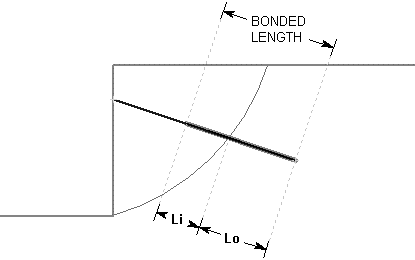Li = bonded length of tieback within sliding mass

Lo = bonded length of tieback embedded beyond slip surface

Tieback Parameters:

B = Bond Strength (force / unit length of bond)

S = Out of Plane Spacing

T = Tensile Capacity (force)

P = Plate Capacity (force)

For a Grouted Tieback, at any point along the length of the tieback, there are 3 possible failure modes which are considered:

1. Pullout (force required to pull the length Lo of the tieback out of the slope)
2. Tensile Failure (maximum axial capacity of the tieback tendon)
3. Stripping (slope failure occurs, but tieback remains embedded in slope)

The maximum force which can be mobilized by each failure mode, PER UNIT WIDTH OF SLOPE, is given by the following equations:

Pullout: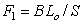Eqn.1

Tensile: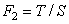Eqn.2

Stripping: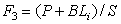Eqn.3

At any point along the length of the tieback, the force which is applied to the slip surface by the tieback, is given by the MINIMUM of these three forces.

Applied Force = min (F1, F2, F3) Eqn.4

• In order for stripping to occur, the Plate Capacity must be exceeded. The Plate Capacity is included in the stripping force equation, and added to the shear capacity along the length Li.
• If the tieback Bond Strength is specified as Material Dependent, then the Pullout Force and Stripping force, are determined by integrating along the bonded length, to determine the force contributed by each segment of the bonded length which passes through different materials.

A Force diagram for a Grouted Tieback, which exhibits all three failure modes, is shown below. In this case, the Plate Capacity is less than the Tensile Capacity, and therefore "stripping" is a possible failure mode. If the Plate Capacity is greater than the Tensile Capacity, then stripping cannot occur, and the Force diagram will be determined only by the Tensile and Pullout failure modes.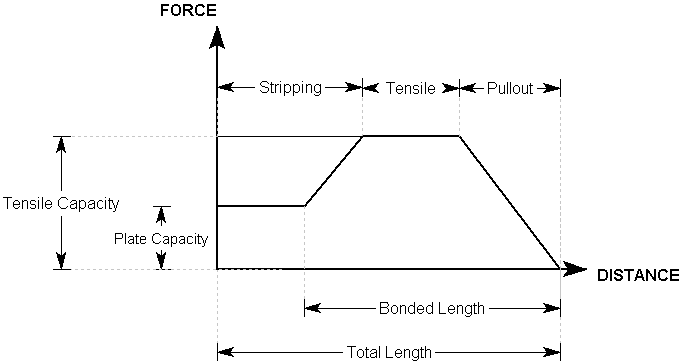Grouted Tieback Force Diagram

Implementation of Shear Capacity

If the Shear Capacity option is selected, an additional force vector perpendicular to the bolt direction, and opposite to the direction of failure, is added to the overall bolt capacity vector, determined by the methods described above. You’ll see this in the Interpreter when you show the slice data. The support force at the base of the slice is no longer parallel to the support but angled in a direction opposite to the slip direction. The Shear Capacity option may be useful if you expect that the support forces are not purely tensile, but may include a transverse shear component as well. This depends on the orientation of the support relative to the slip surface direction.

Illustration of Shear Capacity option for support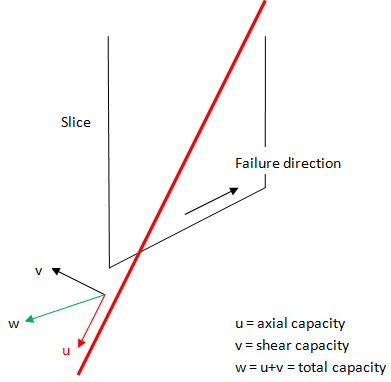Implementation of Compression Capacity

If the Compression Capacity option is selected, the bolt can apply a stabilizing force to the slope when it goes into compression. This can be useful for modelling compression piles, for example. To determine when a bolt goes into compression, the program looks at the direction of displacement at the point where the bolt crosses the failure surface. The direction of displacement is equal to the direction of the base of the slice through which the bolt traverses, as shown in the figure below. If the dot product of vector u and v is positive, the bolt is in compression.

Illustration of Compression Capacity option for supportIf the bolt goes into compression, the capacity is determined in the same way as a bolt-in tension, with the following two differences. The first is the compression capacity is substituted for the tensile capacity. The second is that the plate capacity is assumed to be zero. Three modes of failure are still examined to determine the overall capacity. The first is compression failure of the tendon. The second is the bond failure of the bolt outside the failed mass. The third is the bond failure of the bolt internally to the failed mass. The overall capacity is simply the minimum of these three failure modes, as described above for the tensile failure mode.